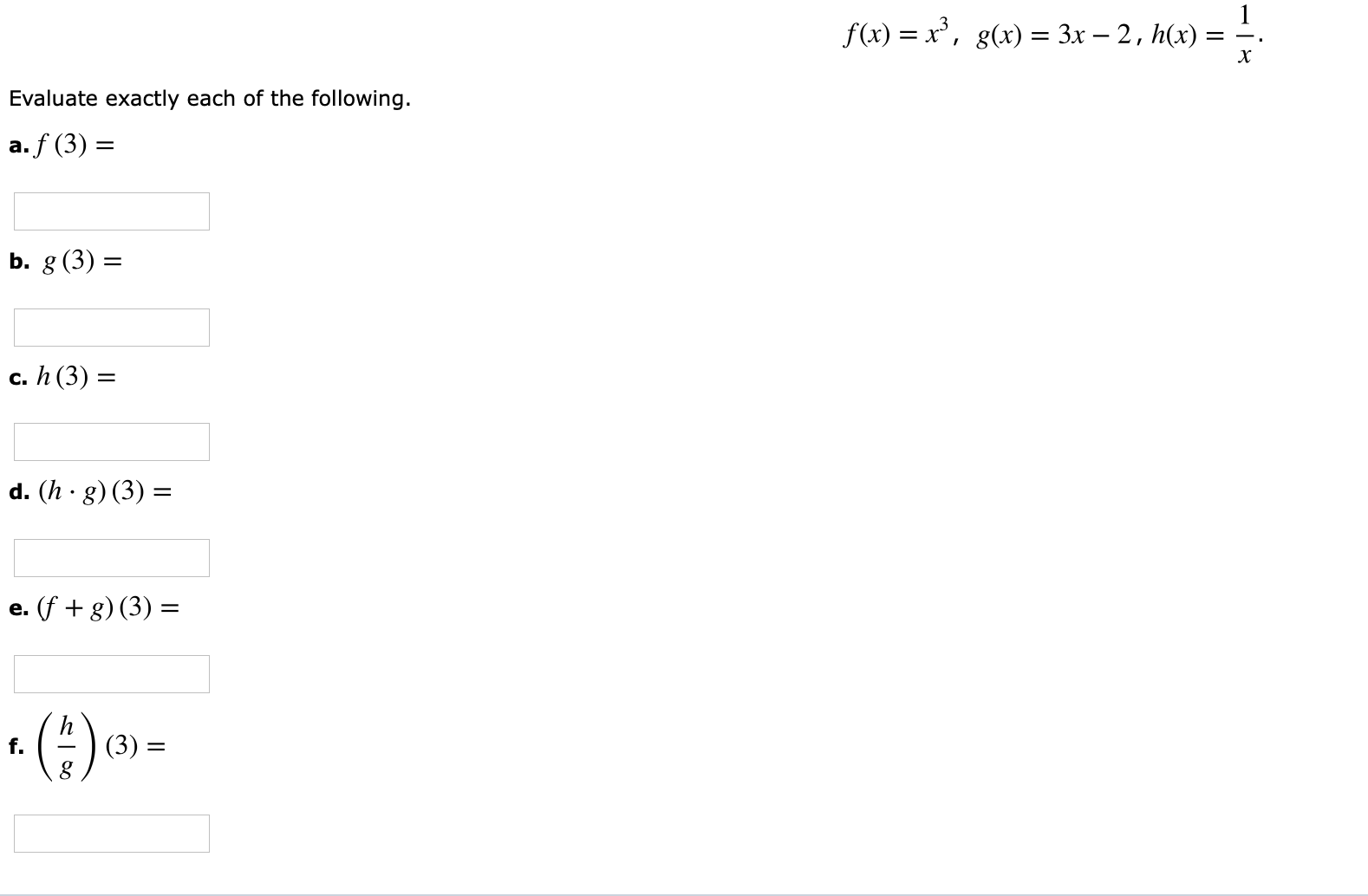Evaluate exactly each of the following.a. f (3)c. h (3)d. (h- g) (3)2f.

Questionhelp_outlineImage TranscriptioncloseEvaluate exactly each of the following. a. f (3) c. h (3) d. (h- g) (3) 2 f. fullscreen
Step 1

Given:

f(x) = x3, g(x) = 3x−2, h(x) = (1/x).

Step 2

Calculation:

a)

Compute the value of f(3) as follows.

Substitute x = 3 in the given f(x) = x3.

f(3) = (3)3

f(3) = 27.

b)

Obtain the value of g(3) by substituting x = 3 in g(x) = 3x−2.

g(3) = 3(3)−2

g(3) = 9−2

g(3) = 7

c)

Evaluate the value of h(3) by substituting  x = 3 in h(x) = (1/x).

Step 3

d)

Compute the value of (h&midd...

Want to see the full answer?

See Solution

Want to see this answer and more?

Our solutions are written by experts, many with advanced degrees, and available 24/7

See Solution
Tagged in

Other